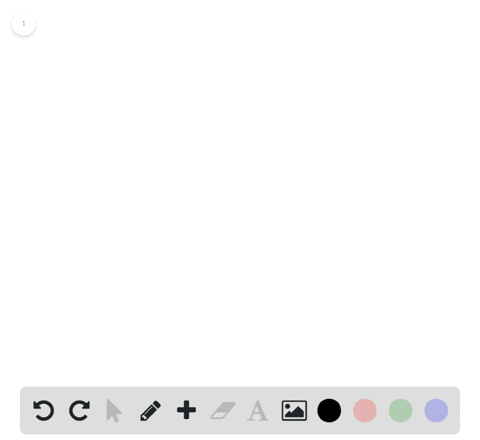Enroll in one of our FREE online STEM bootcamps. Join today and start acing your classes!View Bootcamps00:50
Problem 48

# Using the $\varepsilon$ -\delta Definition of Limit In Exercises $45-56$ , find the limit $L$ . Then use the $\varepsilon-\delta$ definition to prove that the limit is $L$ .$$\lim _{x \rightarrow 3}\left(\frac{3}{4} x+1\right)$$

## Discussion

You must be signed in to discuss.

## Video Transcript

and this problem, we want to find the limit as XO. Purchase three of 3/4 x plus one one. Use that slime Delta definition of limit to find this limit. In other words, you want to show that for every up song grease zero, there exists a delta greater than zero such that the function through X over four plus one, minus 13 over four absolute value is less than epsilon. Whenever X minus three, absolute value is between zero and Delta. No notice that are proposed to limit is 13 over four. This is because for linear functions, which have the form F of X equals MX plus B, the limit as X approaches, si is always going to be, and C equals B, meaning we can just plug in this value were approaching into the function. So also note that if we plug that value in for X, we will get 13 over four. So that's kind of what we proposed that limit. But we need to show this because we're assuming we don't know this claim to be good with. So look at this value here again. So notice that when we simplify this by distributing this negative sign and combining the water Negative three thirteen 13 over four will get three X over four, minus nine over. For I noticed there we can kind of factor out 3/4. So who had the absolute value of 3/4 times X minus tree? We want to factor that out because we want to establish a relationship between this expression and this bound that we have a next my history or down that we have on the absolute value of X fine story. So notice that that term kind of appears here. Further, we can pull out the absolute value of three or four times absolutely of X minus three. And since three over four is already positive, the absolute value of that will just be three or four. So we have three or four times the absolute value of X, my history. So now it seems we should let Delta bi equal to Absalon, which ought to know by the letter a absolute divided by three over fourths, which is the same as four times up Salon over three, and we're done